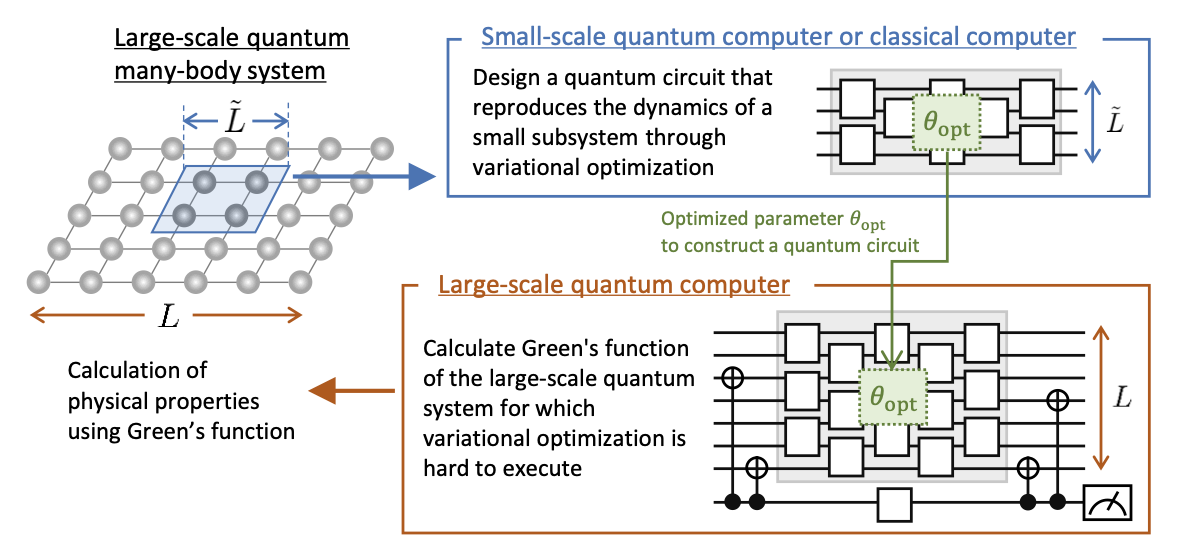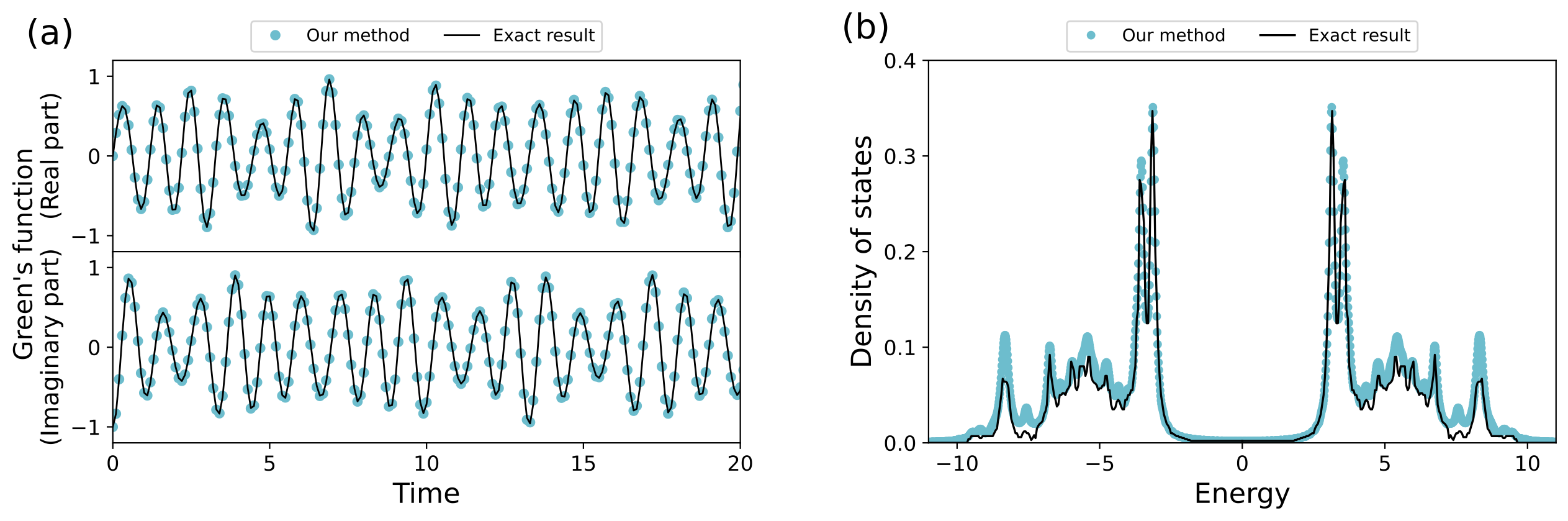News

# Development of an Efficient Method for Computing the Green's Functions of Large-Scale Electronic Systems ~ A Steady Step Toward the Application of Quantum Computers to Strongly-Correlated Electrons ~

QunaSys Inc. (hereafter "QunaSys"), in joint research with Fujitsu Limited (hereafter "Fujitsu"), has developed a method to efficiently compute "Green’s functions"  in large-scale electronic systems using quantum computers. This research result is a steady step toward the realistic application of quantum computers to materials science. The computation of large-scale quantum many-body systems  is one of the most promising areas where quantum computers can realize the computational speedup over classical computers.

The calculation of the Green's function  in electronic systems is extremely important for predicting various physical/chemical properties of matter. A joint research group of QunaSys and Fujitsu has developed a new method for calculating the Green's functions of large-scale electronic systems using a quantum computer by applying the "Local Variational Quantum Compilation (LVQC) method" , which was developed by QunaSys in collaboration with RIKEN and Osaka University in October 2022. The application of LVQC allows us to determine efficient quantum circuits for the computation of large systems by using only optimizations on small quantum systems, so it may be possible to execute it on currently-available noisy intermediate-scale quantum (NISQ) devices and on early fault-tolerant quantum computers (Early-FTQC) .

The research group has illustrated the proposed method numerically and estimated the concrete number of quantum gates and circuit runs required to solve large systems that cannot be solved with a classical computer. Compared to previously proposed methods, the proposed method can compute the Green's function with fewer gates and fewer circuit runs.

The research results were published in Physical Review Research, an online scientific journal of the American Physical Society, on August 1st, 2023, available at https://doi.org/10.1103/PhysRevResearch.5.033070.

Background
Calculating the properties of quantum many-body systems  is considered to be one of the tasks for which quantum computers can outperform classical computers. In particular, elucidating the properties of strongly-correlated electrons, in which electrons in materials strongly interact with each other, is expected to lead to the design of highly functional materials and devices.

In the study of such materials (electronic systems), a quantity called the Green's function of electrons is often calculated. The Green's function is a quantity defined using the dynamics (time-evolution) of electrons and allows the calculation of various physical properties of practical interest, such as the electrical resistance and thermal response of materials.

There have been many proposals for methods to compute the Green's functions using quantum computers, but how to scale up them to large quantum systems has been a challenge. For example, the methods based on applying variational quantum optimization  directly to the target system, which may be feasible for small quantum systems with the current NISQ devices , have a problem of the increasing difficulty and computational cost of optimization for large quantum systems. On the other hand, the Green's function calculation methods based on Trotter decomposition or the quantum singular value transformation  require complex quantum circuits and large-scale fault-tolerant quantum computers, which have not been realized yet.

Methods and Results
In this study, we proposed to apply a method called "Local Variational Quantum Compilation (LVQC)" , developed by QunaSys in 2022 in collaboration with RIKEN and Osaka University, to compute the Green's functions of large-scale electronic systems. LVQC is a method for computing the dynamics of large quantum systems using the results of variational optimization on small quantum systems only and is expected to be feasible using the current NISQ devices and Early-FTQCs.

First, we proved the applicability of LVQC to fermionic systems , including electrons, which was not explicitly considered in the original LVQC proposal. We then proposed an algorithm for computing the Green's function using a quantum circuit that describes the time-evolution determined by LVQC (Figure 1). We have also proposed a method to reduce the computational cost by exploiting the symmetry of the target quantum system.Figure 1: Schematic illustration of the method proposed in this study.

First, a small subsystem is defined in a large-scale quantum many-body system, and a quantum circuit that reproduces its dynamics is designed by variational optimization using a small-scale quantum computer or a classical computer. Next, using the parameters obtained from the variational optimization calculation, a quantum circuit that reproduces the dynamics of the desired large-scale quantum many-body system is efficiently constructed with a small number of quantum gates. Finally, the Green's function is obtained by measuring appropriate expectation values for this quantum circuit.

As a numerical demonstration, we applied the proposed method to a model of strongly-correlated electrons called the Fermi-Hubbard model  in one and two dimensions by simulating the outputs of quantum circuits by a classical computer. The results show that the Green's function and the density of states  in the target large systems can be calculated accurately and efficiently by using the optimization results only on small subsystems (Figure 2). Furthermore, we estimated the number of gates and quantum circuit runs required when the proposed method is applied to the 20 × 20 sites Fermi-Hubbard model, which is difficult to compute an exact solution on a classical computer. It is found that the required resources can be significantly reduced compared to the Trotter decomposition of the conventional method.Figure 2: Numerical illustration of the proposed method.

(a) Time-dependence of the Green's function for the two-dimensional Fermi-Hubbard model on a square lattice of 4 × 2 sites (16 qubits). The blue dots show the results computed by our method, in which the Green's function is computed through variational optimization on a small subsystem of 2 × 2 sites (8 qubits). The solid black line represents the exact result. The results of our method are in good agreement with the exact results on a long time scale.

(b) Density of states for the two-dimensional Fermi-Hubbard model on a square lattice of 4 × 4 sites (32 qubits). The blue dots are the results of our method, calculated using the Green's function obtained through variational optimization on a small subsystem of 2 × 2 sites (8 qubits). The solid black solid line represents the exact calculation results obtained in the previous literature (Leung et al., Physical Review B 46, 11779 (1992).) The results of our method well reproduce the results obtained in the previous literature.

Outlook
The results of this research represent a steady step toward the realistic calculation of the Green's function, one of the most fundamental quantities of quantum many-body systems, using a quantum computer. Since the Green's function is associated with various physical properties, the proposed method has a wide range of possible applications, including the design of the functional materials.
QunaSys and Fujitsu will continue to research and develop practical algorithms for quantum computers and their applications.

Publication Information:
"Computation of Green’s function by local variational quantum compilation",
Shota Kanasugi, Shoichiro Tsutsui, Yuya O. Nakagawa, Kazunori Maruyama, Hirotaka Oshima, and Shintaro Sato
Physical Review Research, 5, 033070 (2023).
https://doi.org/10.1103/PhysRevResearch.5.033070

Footnotes
 Green's function
A quantity used in the theoretical analysis of quantum many-body systems. The Green's function of an electron is defined based on the dynamics (time-evolution) of the electron in the quantum system.

 Quantum many-body system
A physical system in which a large number of particles described by quantum mechanics are. Various phenomena that cannot be seen in classical mechanics occur in this system.

 Local Variational Quantum Compilation (LVQC)
A method for calculating the dynamics of a large-scale quantum system using the results of variational optimization on a small-scale quantum system only, proposed in this paper.

 NISQ device, Early Fault-Tolerant Quantum Computer (Early-FTQC)
NISQ devices, which stands for Noisy Intermidiate-Scale Quantum devices, are quantum computers with tens to thousands of noisy qubits.
The Early-FTQCs are fault-torelant but relatively small-scale quantum computers. Although more qubits and quantum gates are expected to be available in Early-FTQCs than NISQ devices, it will still be impossible to perform calculations to solve large-scale problems that cannot be solved by a classical computer with a naive implementation of existing quantum algorithms.

 Variational quantum optimization
An algorithm that achieves the desired calculation result by variational optimization of a quantum circuit. A typical example of a quantum-classical hybrid algorithm that combines a classical computer with a quantum computer.

 Trotter decomposition and quantum singular value transform
Trotter decomposition is a fundamental method for simulating the time-evolution of a quantum system by a quantum circuit. Quantum singular value transformation is a method that allows various quantum algorithms, including the time-evolution, to be executed in a unified and efficient manner and has attracted much attention in recent years.

 Fermion systems
Particles in the universe are classified into two types of particles with different quantum mechanical behavior: fermions and bosons. For example, electrons are fermions, and photons (quantum particles of light) are bosons.

 Fermi-Hubbard model
One of the most famous physical models for electrons in matter. It can describe various states of matter, such as metals, insulators, and superconductors.

 Density of states
A physical quantity obtained from the Green's function, describing the energy distribution of electrons in a material. It can be used to investigate various physical properties of matter, such as specific heat and electrical conductivity.

Contact
QunaSys PR
pr@qunasys.com

2023/08/04

Category: Press Releases
Category: Press Releases
Year: 2023